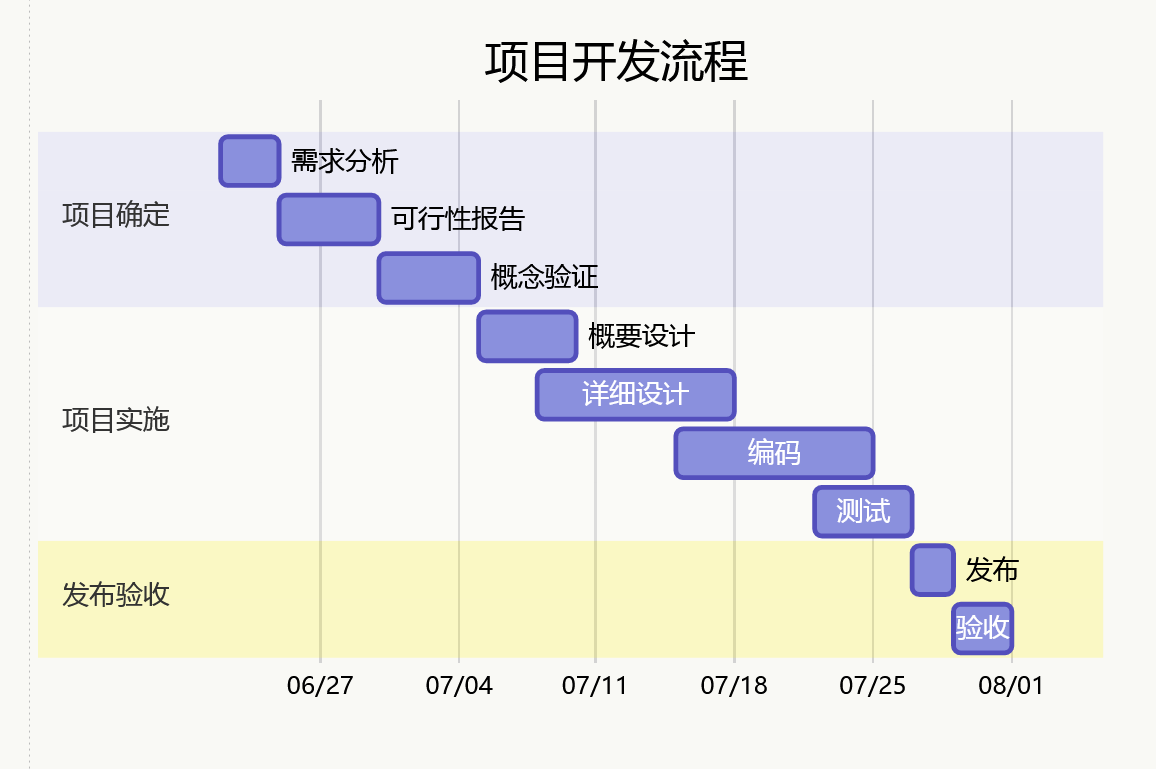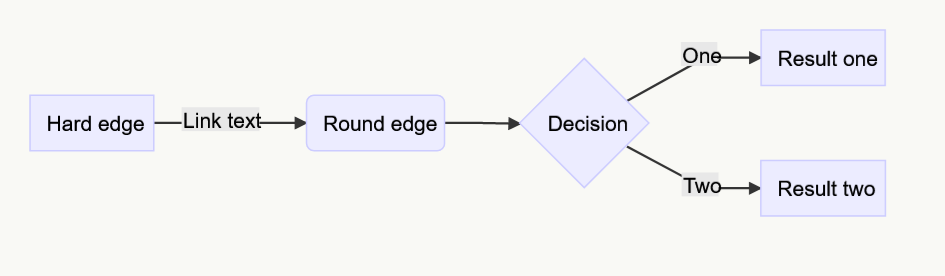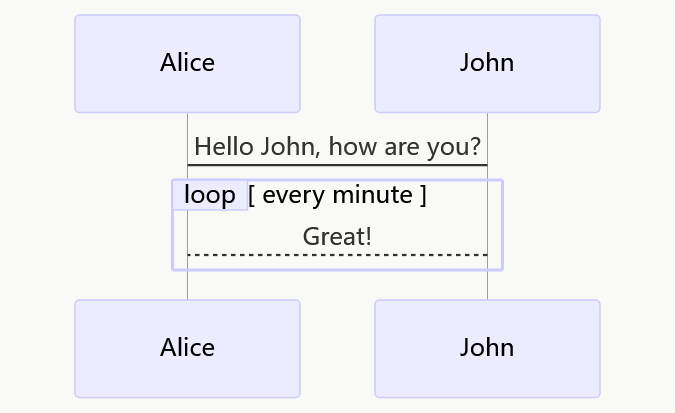### 1. 标签分类

Tags： 数学 英语 Markdown

### 4. LaTeX 公式

$\sum_{i=1}^n a_i=0$

$f(x_1,x_x,\ldots,x_n) = x_1^2 + x_2^2 + \cdots + x_n^2$

$\sum^{j-1}_{k=0}{\widehat{\gamma}_{kj} z_k}$

Python 示例：

JavaScript 示例：

### 8. 甘特图### 9. Mermaid 流程图### 10. Mermaid 序列图### 12. 定义型列表

: 定义 1（左侧有一个可见的冒号和四个不可见的空格）

: 这是代码块的定义（左侧有一个可见的冒号和四个不可见的空格）

    代码块（左侧有八个不可见的空格）


### 13. Html 标签

<table>
<tr>
<th rowspan="2">值班人员</th>
<th>星期一</th>
<th>星期二</th>
<th>星期三</th>
</tr>
<tr>
<td>李强</td>
<td>张明</td>
<td>王平</td>
</tr>
</table>


### 14. 待办事宜 Todo 列表

- [ ] **Cmd Markdown 开发**
- [ ] 改进 Cmd 渲染算法，使用局部渲染技术提高渲染效率
- [ ] 支持以 PDF 格式导出文稿
- [x] 改进 LaTex 功能
- [x] 修复 LaTex 公式渲染问题
- [x] 新增 LaTex 公式编号功能 [语法参考](http://docs.mathjax.org/en/latest/tex.html#tex-eq-numbers)
- [ ] **七月旅行准备**
- [ ] 准备邮轮上需要携带的物品
- [ ] 浏览日本免税店的物品
- [x] 购买蓝宝石公主号七月一日的船票


• [ ] Cmd Markdown 开发
• [ ] 改进 Cmd 渲染算法，使用局部渲染技术提高渲染效率
• [ ] 支持以 PDF 格式导出文稿
• [x] 新增Todo列表功能 语法参考
• [x] 改进 LaTex 功能
• [x] 修复 LaTex 公式渲染问题
• [x] 新增 LaTex 公式编号功能 语法参考
• [ ] 七月旅行准备
• [ ] 准备邮轮上需要携带的物品
• [ ] 浏览日本免税店的物品
• [x] 购买蓝宝石公主号七月一日的船票

: 这是一个 注脚文本

: 这是另一个 注脚文本